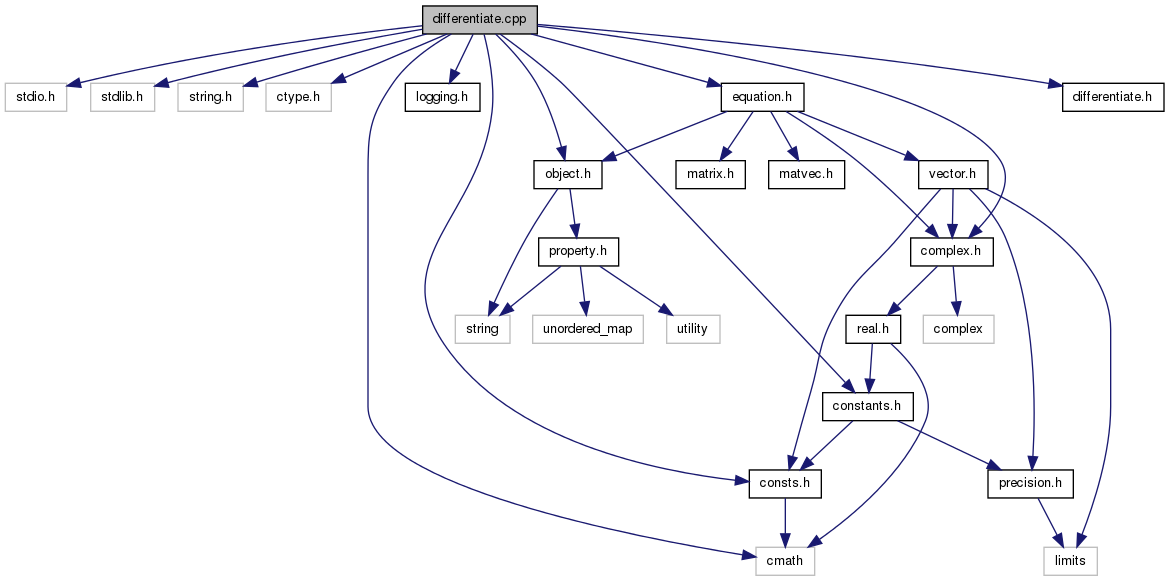Qucs-core  0.0.19
differentiate.cpp File Reference
`#include <stdio.h>`
`#include <stdlib.h>`
`#include <string.h>`
`#include <ctype.h>`
`#include <cmath>`
`#include "logging.h"`
`#include "complex.h"`
`#include "object.h"`
`#include "consts.h"`
`#include "equation.h"`
`#include "differentiate.h"`
`#include "constants.h"`
Include dependency graph for differentiate.cpp:Go to the source code of this file.

struct  eqn

## Defines

#define C(con)   ((constant *) (con))
#define A(con)   ((application *) (con))
#define R(con)   ((reference *) (con))
#define D(con)   (C(con)->d)
#define isConst(n)   ((n)->getTag()==CONSTANT && C(n)->getType()==TAG_DOUBLE)
#define isRef(r, v)   ((r)->getTag()==REFERENCE && !strcmp(R(r)->n,v))
#define isZero(n)   (isConst(n) && D(n) == 0.0)
#define isOne(n)   (isConst(n) && D(n) == 1.0)
#define isNeg(n)   (isConst(n) && D(n) == -1.0)
#define isEuler(n)   ((isConst(n) && D(n) == euler) || isRef(n,"e"))
#define isval(n, v)   (isConst(n) && D(n) == v)
#define isVar(v)   ((v)->getTag()==REFERENCE)
#define isApp(v)   ((v)->getTag()==APPLICATION)
#define isMul(v)   (isApp(v) && !strcmp(A(v)->n,"*"))
#define isSqr(v)   (isApp(v) && !strcmp(A(v)->n,"sqr"))
#define retCon(val)   constant * res = new constant (TAG_DOUBLE); res->d = val; return res;
#define defCon(def, val)   constant * def = new constant (TAG_DOUBLE); def->d = val;
#define defRef(def, var)   reference * def = new reference (); def->n = strdup (var);
#define retApp1(op, f0)
#define defApp1(def, op, f0)
#define defApp2(def, op, f0, f1)
#define retApp2(op, f0, f1)
#define retApp3(op, f0, f1, f2)
#define defApp3(def, op, f0, f1, f2)
#define _A(idx)   app->args->get(idx)
#define _A0   _A(0)
#define _A1   _A(1)
#define _A2   _A(2)
#define _D0   _A(0)->differentiate (derivative)
#define _D1   _A(1)->differentiate (derivative)
#define _D2   _A(2)->differentiate (derivative)
#define _AF0(var)   node * var = _A0;
#define _AF1(var)   node * var = _A1;
#define _AF2(var)   node * var = _A2;
#define _AD0(var)   node * var = _D0;
#define _AD1(var)   node * var = _D1;
#define _AD2(var)   node * var = _D2;
#define _AA(a, idx)   A(a)->args->get(idx)
#define _AA0(a)   _AA(a,0)
#define _AA1(a)   _AA(a,1)
#define _AAF0(a, var)   node * var = _AA0(a);
#define _AAF1(a, var)   node * var = _AA1(a);

## Define Documentation

 #define _A ( idx ) app->args->get(idx)

Definition at line 91 of file differentiate.cpp.

 #define _A0   _A(0)

Definition at line 92 of file differentiate.cpp.

 #define _A1   _A(1)

Definition at line 93 of file differentiate.cpp.

 #define _A2   _A(2)

Definition at line 94 of file differentiate.cpp.

 #define _AA ( a, idx ) A(a)->args->get(idx)

Definition at line 106 of file differentiate.cpp.

 #define _AA0 ( a ) _AA(a,0)

Definition at line 107 of file differentiate.cpp.

 #define _AA1 ( a ) _AA(a,1)

Definition at line 108 of file differentiate.cpp.

 #define _AAF0 ( a, var ) node * var = _AA0(a);

Definition at line 110 of file differentiate.cpp.

 #define _AAF1 ( a, var ) node * var = _AA1(a);

Definition at line 111 of file differentiate.cpp.

 #define _AD0 ( var ) node * var = _D0;

Definition at line 102 of file differentiate.cpp.

 #define _AD1 ( var ) node * var = _D1;

Definition at line 103 of file differentiate.cpp.

 #define _AD2 ( var ) node * var = _D2;

Definition at line 104 of file differentiate.cpp.

 #define _AF0 ( var ) node * var = _A0;

Definition at line 99 of file differentiate.cpp.

 #define _AF1 ( var ) node * var = _A1;

Definition at line 100 of file differentiate.cpp.

 #define _AF2 ( var ) node * var = _A2;

Definition at line 101 of file differentiate.cpp.

 #define _D0   _A(0)->differentiate (derivative)

Definition at line 95 of file differentiate.cpp.

 #define _D1   _A(1)->differentiate (derivative)

Definition at line 96 of file differentiate.cpp.

 #define _D2   _A(2)->differentiate (derivative)

Definition at line 97 of file differentiate.cpp.

 #define A ( con ) ((application *) (con))

Definition at line 47 of file differentiate.cpp.

 #define C ( con ) ((constant *) (con))

Definition at line 46 of file differentiate.cpp.

 #define D ( con ) (C(con)->d)

Definition at line 49 of file differentiate.cpp.

 #define defApp1 ( def, op, f0 )
Value:
```application * def = new application (); def->n = strdup (op); \
def->nargs = 1; def->args = f0; def->args->setNext (NULL);
```

Definition at line 73 of file differentiate.cpp.

 #define defApp2 ( def, op, f0, f1 )
Value:
```application * def = new application (); def->n = strdup (op); \
def->nargs = 2; def->args = f0; def->args->append (f1);
```

Definition at line 76 of file differentiate.cpp.

 #define defApp3 ( def, op, f0, f1, f2 )
Value:
```application * def = new application (); def->n = strdup (op); \
def->nargs = 3; def->args = f0; def->args->append (f1); \
def->args->append (f2);
```

Definition at line 86 of file differentiate.cpp.

 #define defCon ( def, val ) constant * def = new constant (TAG_DOUBLE); def->d = val;

Definition at line 66 of file differentiate.cpp.

 #define defRef ( def, var ) reference * def = new reference (); def->n = strdup (var);

Definition at line 68 of file differentiate.cpp.

 #define isApp ( v ) ((v)->getTag()==APPLICATION)

Definition at line 60 of file differentiate.cpp.

 #define isConst ( n ) ((n)->getTag()==CONSTANT && C(n)->getType()==TAG_DOUBLE)

Definition at line 51 of file differentiate.cpp.

 #define isEuler ( n ) ((isConst(n) && D(n) == euler) || isRef(n,"e"))

Definition at line 56 of file differentiate.cpp.

 #define isMul ( v ) (isApp(v) && !strcmp(A(v)->n,"*"))

Definition at line 61 of file differentiate.cpp.

 #define isNeg ( n ) (isConst(n) && D(n) == -1.0)

Definition at line 55 of file differentiate.cpp.

 #define isOne ( n ) (isConst(n) && D(n) == 1.0)

Definition at line 54 of file differentiate.cpp.

 #define isRef ( r, v ) ((r)->getTag()==REFERENCE && !strcmp(R(r)->n,v))

Definition at line 52 of file differentiate.cpp.

 #define isSqr ( v ) (isApp(v) && !strcmp(A(v)->n,"sqr"))

Definition at line 62 of file differentiate.cpp.

 #define isval ( n, v ) (isConst(n) && D(n) == v)

Definition at line 57 of file differentiate.cpp.

 #define isVar ( v ) ((v)->getTag()==REFERENCE)

Definition at line 59 of file differentiate.cpp.

 #define isZero ( n ) (isConst(n) && D(n) == 0.0)

Definition at line 53 of file differentiate.cpp.

 #define R ( con ) ((reference *) (con))

Definition at line 48 of file differentiate.cpp.

 #define retApp1 ( op, f0 )
Value:
```application * res = new application (); res->n = strdup (op); \
res->nargs = 1; res->args = f0; res->args->setNext (NULL); return res;
```

Definition at line 70 of file differentiate.cpp.

 #define retApp2 ( op, f0, f1 )
Value:
```application * res = new application (); res->n = strdup (op); \
res->nargs = 2; res->args = f0; res->args->append (f1); return res;
```

Definition at line 79 of file differentiate.cpp.

 #define retApp3 ( op, f0, f1, f2 )
Value:
```application * res = new application (); res->n = strdup (op); \
res->nargs = 3; res->args = f0; res->args->append (f1); \
res->args->append (f2); return res;
```

Definition at line 82 of file differentiate.cpp.

 #define retCon ( val ) constant * res = new constant (TAG_DOUBLE); res->d = val; return res;

Definition at line 64 of file differentiate.cpp.